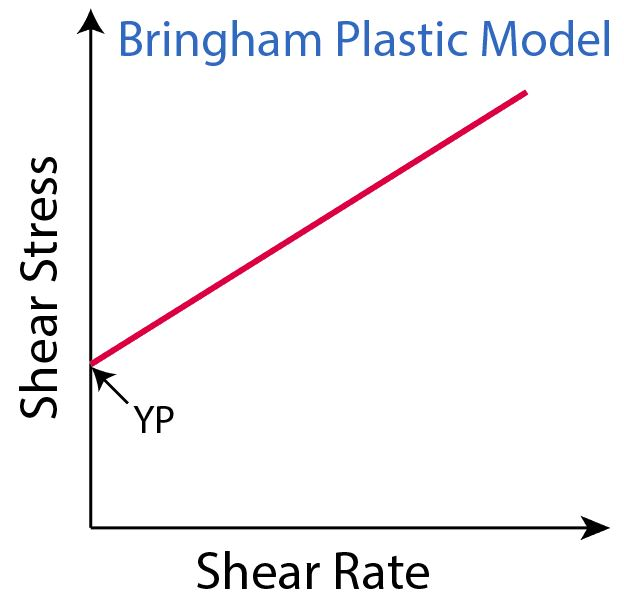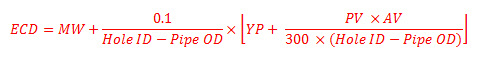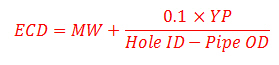## Yield Point (YP) of Drilling FluidsYield Point (YP) is resistance of initial flow of fluid or the stress required in order to move the fluid. It can be simply stated that the Yield Point (YP) is the attractive force among colloidal particles in drilling fluid. As per Bingham plastic model, YP is the shear stress extrapolated to a shear rate of zero.Figure 1 – YP is a shear stress at zero shear rate. Continue reading

## Equivalent Circulating Density (ECD) Using Yield Point for MW More than 13 ppg

Another equation to determine equivalent circulating density is to use yield point.This formula below is used to calculate the ECD and it is good for mud weight more than to 13.0 ppgWhere:

ECD is equivalent circulating density in ppg.

MW is mud weight in ppg.

Hold ID is inside diameter of hole in inch.

Pipe OD is outside diameter of pipe in inch.

YP is mud yield point.

YP can be calculated by these following equations.

YP = Reading at 300 rpm – PV

AV is annular velocity in ft/min

AV can be determined by the following equation.

AV in ft/min = (24.5 x Q) ÷ (Dh2 – Dp2)

where

Q = flow rate in gpm

Dh = inside diameter of casing or hole size in inch

Dp = outside diameter of drill pipe, drill collars, or tubing in inch

## Equivalent Circulating Density (ECD) Using Yield Point for MW less than 13 ppg

Another way to determine equivalent circulating density (ECD) is to use yield point.This formula below is used to calculate the ECD and it is good for mud weight less than or equal to 13.0 ppg.Where:

ECD is equivalent circulating density in ppg

MW is mud weight in ppg

Hold ID is inside diameter of hole in inch

Pipe OD is outside diameter of pipe in inch

YP is mud yield point

YP can be calculated by these following equations

YP = Reading at 300 rpm – PV